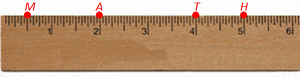# Ruler Postulate: Definition & Examples

Instructions:

Choose an answer and hit 'next'. You will receive your score and answers at the end.

question 1 of 3

### The ruler postulate uses which formula to find the distance between two points?

Create Your Account To Take This Quiz

As a member, you'll also get unlimited access to over 75,000 lessons in math, English, science, history, and more. Plus, get practice tests, quizzes, and personalized coaching to help you succeed.

Try it risk-free for 30 days. Cancel anytime.

### 1. Find the distance between point M and H.### 2. Find the distance between point T and A.Create your account to access this entire worksheet
A Premium account gives you access to all lesson, practice exams, quizzes & worksheets
Quizzes, practice exams & worksheets
Certificate of Completion
Create an account to get started

A series of questions (most of which are example problems) will primarily prompt you to display what you do and don't know when it comes to the distances between points.

## Quiz & Worksheet Goals

To be specific, know you'll demonstrate your understanding of:

• How the ruler postulate formula works
• When you should use the ruler postulate formula
• What the ruler postulate formula calculates
• How you can find the distance between two points

## Skills Practiced

• Problem solving - use acquired knowledge to solve problems on finding the distance between points
• Knowledge application - use your knowledge to answer questions by using the ruler postulate formula
• Reading comprehension - ensure that you draw the most important information from the related ruler postulate lesson

Advance your knowledge of the ruler postulate by making use of our lesson by the name, Ruler Postulate: Definition & Examples. This particular lesson will aid you with information concerning:

• The way the ruler postulate works
• Distance measurement via the ruler postulate
• Roles of the ruler postulate
• Examples of how the ruler postulate works
Final ExamSAT Mathematics Level 2: Help and Review
Status: Not Started
Chapter ExamBasic Math Review: Help and Review
Status: Not Started

Support